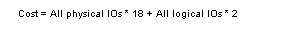# Total actual I/O cost value

If resource limits are enabled, statistics io prints the “Total actual I/O cost” line. Adaptive Server reports the total actual I/O as a unitless number. The formula for determining the cost of a query is:This formula multiplies the “cost” of a logical I/O by the number of logical I/Os and the “cost” of a physical I/O by the number of physical I/Os.

For the example above that performs 656 physical reads and 656 logical reads, 656 * 2 + 656 * 18 = 13120, which is the total I/O cost reported by statistics io.